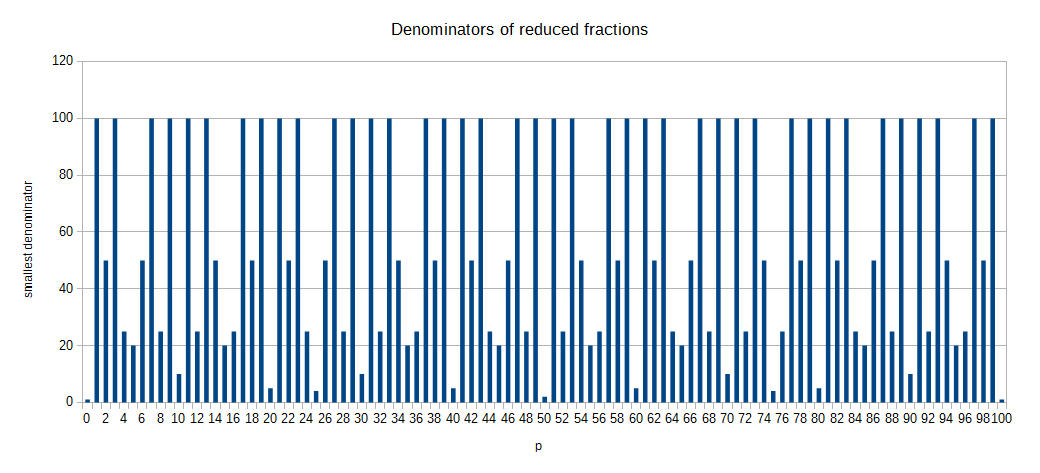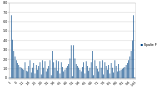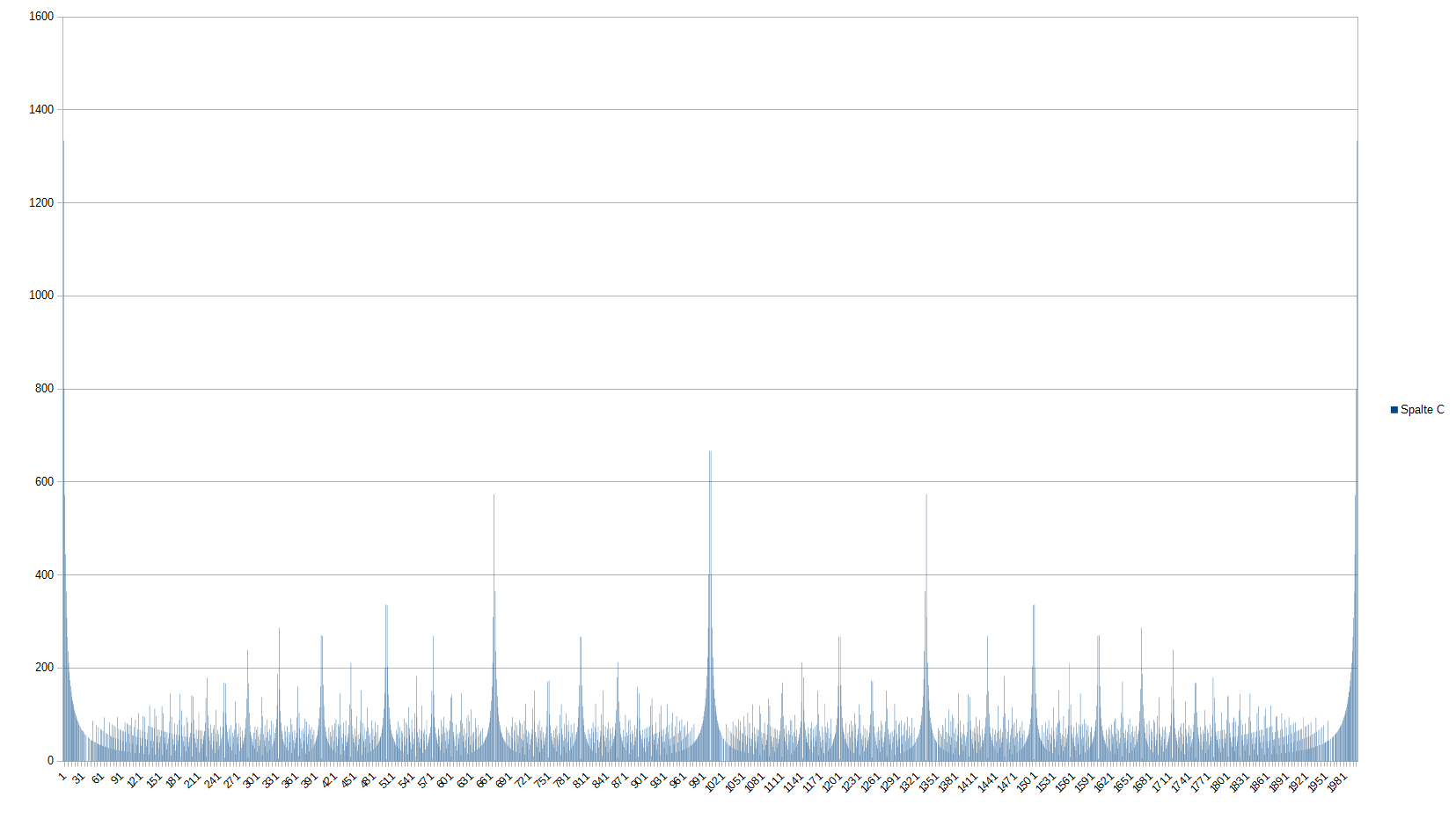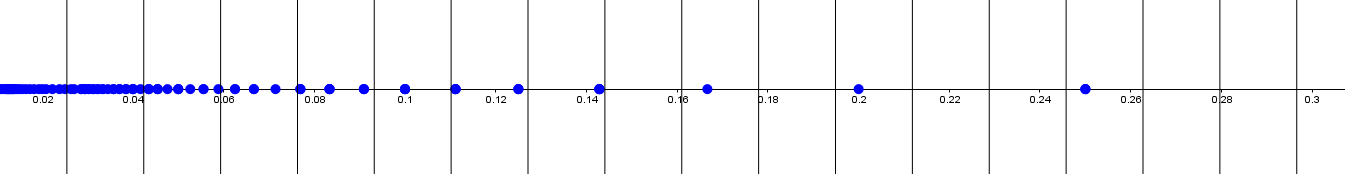# Percentages and decimals as rounded fractions

Related problem

Of course, every possible integer percentage $0 \leq p \leq 1, 100p \in \mathbb{N}$ can be expressed as a fraction $\frac a {100}, a=100p$. Many of these fractions (actually 61, because $61 = 101 - \phi(100) = 101 - 40$ with Euler's totient function $\phi(n)$) can be reduced to have a smaller denominator.However, if we allow rounding, we can express most percentages with even smaller denominators, e.g. $17\% \approx \frac 1 6$.

Rounding

First of all, we have to define how to round. There are many ways to do this, but we have to conditions that characterize the method we'll use.

• Every number $p$ that isn't already an integer (in this case we wouldn't have to round) has two neighbouring integers. Unless the fractional part of $p$, $p-\lfloor p \rfloor$ is $0.5$ we can just round to the nearest integer.
• When working with fractions, there is a nice symmetry $\frac a b + \frac {b-a} b = 1$. This seems trivial, but we definitely want to keep it. So $\frac 1 8 + \frac 7 8$ should equal $1$. But when rounded, we get $0.13 + 0.88 = 1.01$. So, to preserve symmetry, we will always round away from $0.5$. $0.125 ≈ 0.12$, but $0.875 ≈ 0.88$.

These two conditions characterize our rounding method, and we can also write a formula that satisfies both:

$p \approx \frac 1 {100} ( \text{sgn} (100p-50) \lfloor | 100p-50 | + \frac1 2 \rfloor +50 )$

The sign-function $\text{sgn} (x)$ gives $1$ if $x$ is nonnegative and $-1$ otherwise.

If we now want to round with some other precision $a \in \mathbb{N}$, we can use the formula

$p \stackrel{s}\approx \frac 1 s \left( \text{sgn} (sp-\frac s 2) \lfloor | sp-\frac s 2 | + \frac1 2 \rfloor + \frac s 2 \right)$

To give another notation, let's define

$r_s(p) = \frac 1 s \left( \text{sgn} (sp-\frac s 2) \lfloor | sp-\frac s 2 | + \frac1 2 \rfloor + \frac s 2 \right)$

and in particular

$r(p) = r_{100}(p)$

The smallest possible denominator

With these rules in mind, we can now look at the topic of this note – expressing decimals (like percentages) as rounded fractions.

As common, we will only consider reduced fractions; and in this part mainly their denominators.

Let us define a function $q_s(p)$ to give the smallest denominator $q$ for which there exists a numerator $a$ so that $\frac a q$ equals $p$ when rounded with precision $s$.

$q_s(p) = \min \{q \in \mathbb{N} : (\exists a \in \mathbb{N})[r_s(\frac a q) = p] \}$

Now we can explore some properties of $q_s(p)$.

If we graph $q_s(p)$ with some fixed precision, say $s=100$, we see some interesting patterns.

Every $p$ that can be written with a small denominator $q$ has neighbours that need very large denominators. For example, $p=0.5 = \frac 1 2 \Rightarrow q_{100}(0.5)=2$, but $q_{100}(0.49) = 35$. This is because for every other $q < 35$, either the fraction could be reduced to exactly $\frac 1 2$ or $\frac 1 q$ just didn't make small enough steps to hit $0.49$.

We can make a table of $q_s(p)$ when $s$ goes to infinity and $p$ is very small, so it's influenced by $0$.

$\displaystyle \lim_{s \to \infty}q_s(1) = \left\lceil \frac 2 3 s \right\rceil$

$\displaystyle \lim_{s \to \infty}q_s(2) = \left\lceil \frac 2 5 s \right\rceil$

And in general,

$\displaystyle \lim_{s \to \infty}q_s(p) = \left\lceil \frac 2 {2p+1} s \right\rceil$

Influence

To formalize this influence of simple fractions, let's define a funtion $i_s(p)$ to give the number of values from $p$ upward for which $q$ is decreasing.

$i_s(p) = max\{ p_k : sp_k \in \mathbb{N} \land q_s\left(p+\frac 1 s\right)>q_s\left(p+\frac 2 s\right)>\cdots>q_s\left(p+\frac k s\right) \} - p$

We can look at another diagram of $q_s(p)$ with bigger $s$, in this case $s=2000$) to see how $i_s(p)$ behaves.There are all those spikes marking the neighbours of simple fractions, and it somehow looks a little bit like a fractal.

The influence of $0$ is easiest to calculate. It can be done with a computer program, but there is no closed formula since it really depends on the value of $s$.

pseudocode:

 1 2 3 4 5 6 if (x=0) (f(1/s, s)) else if (1/floor(1/x)>x+1/(2*s) and 1/ceiling(1/x)<=x-1/(2*s)) x else f(x+1/s, s) 

What we can do, is give an approximation for $i(0, s)$ when $s$ gets very large. Since the influence counts the number of $p$-values whose denominators are decreasing, it will stop at the first fraction with a higher denominator, say $p_1$. But the one before it, $p_0$, had a smaller denominator and was smaller. So it necessarily also had a smaller numerator, actually $1$ because otherwise it could have been replaced by some other fraction with numerator $1$ and smaller denominator. From all this we get that the first fraction $p_1$ to interrup the sequence has to have a numerator greater than $1$. This means that there is no fraction with numerator $1$ that would get rounded to $p_1$, so it's too far away from any unit fraction.

We can imagine regions in which all numbers get rounded to the same number as a grid with each cell spanning $\frac 1 s$ units.This is the grid for $s=59$. Every point marks one unit fraction and will get rounded to the center of the interval it is in. We notice that between $0.14$ and $0.16$ there is an empty interval. This corresponds to the fact that no unit fraction will get rounded to approximately $0.15$$\frac 1 6$ is too big and $\frac 1 7$ too small. So actually, we just have to find the first interval without any unit fractions and then we can calculate $i(0, s)$.

Finding the empty interval

To find an empty interval, we have to compare the size of the intervals (which is constant and equals $\frac 1 s$) with the size of the gaps between unit fractions.

The difference between some unit fraction $\frac 1 n$ and the next one, $\frac 1 {n+1}$ is given by

$\frac 1 n - \frac 1 {n+1} = \frac 1 {n(n+1)} = \frac 1 {n^2+n}$

If this gap is greater than $\frac 1 s$, this doesn't imply that the interval is empty. $\frac 1 n$ could still be in the interval, $\frac 1 {n+1}$ in the previous and $\frac 1 {n-1}$ in the next.

What is sufficient, is that the gap is greater than $\frac 2 s$. A unit fraction could still be in this interval, but either the previous or the next have to leave an empty interval. To find an $n$ that satisfies this, we set up the inequality

\begin{aligned} \frac 1 {n^2+n} &\geq \frac 2 s \\ n^2+n &\leq \frac s 2 \\ n^2+n-\frac 1 2 s &\leq 0 \\ n &\leq \frac {-1 \pm \sqrt{1+2s}}{2} \\ n &\leq \frac {\sqrt{2s+1}-1}{2} \end{aligned}

At first this looks like an upper bound, but actually it is our lower bound for $n$ or upper bound for $\frac 1 n$. This is because the inequality gives us a sufficient condition, so there could be smaller poasible values for $n$. We have to remember that and use it later. Now, we also have to find a upper bound for $n$.

If the gap between two unit fractions is less than $\frac 1 s$, the spacing of our intervals, then there has to be a unit fraction in every interval. So we can set up a second inequality

\begin{aligned} \frac 1 {n^2+n} &< \frac 1 s \\ n^2+n &> s \\ n^2+n-s &> 0 \\ n &> \frac {-1 \pm \sqrt{1+4s}}{2} \\ n &> \frac {\sqrt{4s+1}-1}{2} \\ \end{aligned}

This is also actually an upper bound, because it is a sufficient condition that there is nos empty interval for all $n$ values. Ther could be a gap for $n$ greater than that.

If we now combine the two, rembering to swap the inequality signs, we find the following conditions for $n$

$\frac {\sqrt{4s+1}-1}2 \geq n > \frac{\sqrt{2s+1}-1}2$

Somewhere in this region there will be some $n$ that works. When $s$ gets very large, we can say

$\frac {\sqrt{4s+1}-1}2 \sim \sqrt{s}$

and

$\frac {\sqrt{2s+1}-1}2 \sim \frac 1 {\sqrt{2}} \sqrt{s}$

But, for the influence we care about $\frac 1 n$ and we have to multiply by $s$ to get the number of influenced $p$s, not the value. So we see, when $s$ is large,

$\sqrt{s} < i(0, s) < \sqrt{2}\sqrt{s}$

Variables and functions I used

Variables

• $a, b, c, d \in \mathbb{N}$: temporary variables
• $k$: some index
• $0 \leq p \leq 1, p \in \mathbb{R}$: the value we want to express
• $s \in \mathbb{N}$: the precision of our rounding
• $q \in \mathbb{N}$: the smallest possible denominator
• $i, j$: the influence

Functions

• $\lfloor \cdot \rfloor$: The floor function
• $\text{sgn} (\cdot)$: The sign function
• $|\cdot|$: the absolute valueNote by Henry U
2 years, 9 months ago

This discussion board is a place to discuss our Daily Challenges and the math and science related to those challenges. Explanations are more than just a solution — they should explain the steps and thinking strategies that you used to obtain the solution. Comments should further the discussion of math and science.

When posting on Brilliant:

• Use the emojis to react to an explanation, whether you're congratulating a job well done , or just really confused .
• Ask specific questions about the challenge or the steps in somebody's explanation. Well-posed questions can add a lot to the discussion, but posting "I don't understand!" doesn't help anyone.
• Try to contribute something new to the discussion, whether it is an extension, generalization or other idea related to the challenge.

MarkdownAppears as
*italics* or _italics_ italics
**bold** or __bold__ bold
- bulleted- list
• bulleted
• list
1. numbered2. list
1. numbered
2. list
Note: you must add a full line of space before and after lists for them to show up correctly
paragraph 1paragraph 2

paragraph 1

paragraph 2

[example link](https://brilliant.org)example link
> This is a quote
This is a quote
    # I indented these lines
# 4 spaces, and now they show
# up as a code block.

print "hello world"
# I indented these lines
# 4 spaces, and now they show
# up as a code block.

print "hello world"
MathAppears as
Remember to wrap math in $$ ... $$ or $ ... $ to ensure proper formatting.
2 \times 3 $2 \times 3$
2^{34} $2^{34}$
a_{i-1} $a_{i-1}$
\frac{2}{3} $\frac{2}{3}$
\sqrt{2} $\sqrt{2}$
\sum_{i=1}^3 $\sum_{i=1}^3$
\sin \theta $\sin \theta$
\boxed{123} $\boxed{123}$

Sort by:

Henry, you switched the bracket signs for the links and AWESOME NOTE!!!!

- 2 years, 9 months ago

Thank you!! It's my first note of this kind and I'm glad you like it.

- 2 years, 9 months ago

Actually, if you progress at this rate, YOU ARE A LEGEND!

- 2 years, 9 months ago

Thank you SOO much!!

- 2 years, 9 months ago

You put in so much effort and you dare say that this is your first note! Imagine you are a seasoned note writer! LEGEND!

- 2 years, 9 months ago

Does anyone know how to define a function $i(p, s)$ in real code, maybe in Python?

 if (p=0) then (i(1/s, s)) else if (1/floor(1/p)>p+1/(2*s) and 1/ceiling(1/p)<=x-1/(2*s)) then p else i(p+1/s, s)

- 2 years, 9 months ago